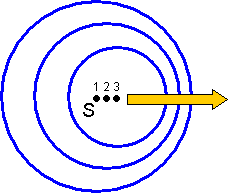##### Doppler effect (1)
The Doppler effect is the apparent change of frequency and wavelength when a source of waves and an observer move relative to each other.###### A source S moves from left to right. Initially it is at position 1 and some time later at positions 2 and 3. If it is emitting a wave then the three circles represent the positions of the waves emitted at points 1, 2 and 3 some time after the source passed position 3. The wavelengths on the right are closer together than those on the left; if the source is approaching an observer the wavelength will be reduced while if it is moving away they will be increased.
 New wavelength (lĺ) = l[1 ▒ v/c] and Wavelength change (Dl) = lv/c These two formulae are valid for both electromagnetic radiation and sound. New frequency (fĺ) = f[1 -/+ v/c] and Frequency change (Df) = fv/c These two formulae are valid for both electromagnetic radiation but NOT for sound.